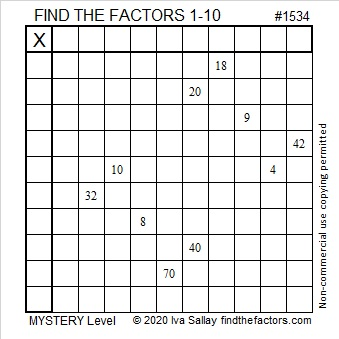# 1534 Dem Bones

### Today’s Puzzle:

You’ve probably heard someone sing about Dem Bones before. It is a song that describes how bones in the body are connected to each other. Ezekiel 37:1-14 was the inspiration for the lyrics, and the song is the inspiration for today’s mystery level puzzle:### Factors of 1534:

• 1534 is a composite number.
• Prime factorization: 1534 = 2 × 13 × 59.
• 1534 has no exponents greater than 1 in its prime factorization, so √1534 cannot be simplified.
• The exponents in the prime factorization are 1, 1, and 1. Adding one to each exponent and multiplying we get (1 + 1)(1 + 1)(1 + 1) = 2 × 2 × 2 = 8. Therefore 1534 has exactly 8 factors.
• The factors of 1534 are outlined with their factor pair partners in the graphic below.### More Facts about the Number 1534:

1534 is the hypotenuse of a Pythagorean triple:
590-1416-1534 which is (5-12-13) times 118.

1534 is 4(7³) + 3(7²) + 2(7¹) + 1(7º). That means it is 4321 in base 7.